Introduction

GPrank package has been built upon the gptk package implemented by Kalaitzis et al., 2011 with the addition of “fixed variance”" kernel which allows to incorporate additional variance information from pre-processing of the observations into the Gaussian process (GP) regression models.

GPs are an ideal model for short and irregularly sampled time series and they can be used to model and rank multiple time series, each generated by different items within an experiment.

In our papers Topa et al., 2015 and Topa and Honkela, 2016, we have proposed variance estimation methods for Pool-seq and RNA-seq data, and evaluated the performance of our GP-based ranking method by comparing the precision and recall under different scenarios with and without variance usage. Simulation results have shown that variance usage leads to a higher average precision, which means less false positives appearing in the top of the ranked list. Motivated by these results, here we will explain how to use GPrank package and then provide examples for two different applications we had in our papers.

Citing GPrank

To cite GPrank in publications, please cite relevant of the two methodology papers Topa et al., 2015 and Topa and Honkela, 2016 that the software is based on.

Methods overview

Our GP-based ranking method uses Bayes factors to rank muliple time series where the Bayes factors are computed for each item by the ratio of its maximum marginal likelihood under two alternative GP models, namely time-dependent and time-independent. Time-independent model (which is referred as the null model in the package) assumes no temporal dependency between observations and uses only a white noise kernel to model the noise. Time-dependent model (which is referred as the model in the package) on the other hand, assumes a smooth temporal behavior, and in addition to the white noise kernel, it also includes a radial basis function (RBF) kernel to capture the temporal dependency. Furthermore, we use a fixed variance kernel in both models in order to incorporate variance information which could be obtained by appropriate estimation methods during pre-processing. For more technical details about the GP models, please refer to Rasmussen and Williams, 2006 as well as the papers mentioned in section Citing GPrank.

General usage of GPrank

Installing the package

In order to install GPrank package from the GitHub repository, start R and run the following command:

In order to install from CRAN, simply use the following command:

Necessary data

In order to construct a GP model, three vectors must be provided for each item. These vectors are:

• t: vector containing the input values, i.e., sampled time points.

• y: vector containing the observed values at the corresponding time points in vector t.

• v: vector containing the variances at the corresponding time points in vector t.

Once we have obtained these vectors, we can construct a GP model with constructModel function using different kernels such as ‘rbf’, ‘white’, and ‘fixedvariance’.

Example:

Please make sure that the three vectors have the same length with each other. If the data is replicated, please remember to adjust the input vector accordingly. For example, if there are two replicates observed at n time points from time t1 to tn, vector t must be defined as: t=[t1, t1, t2, t2, … , tn, tn].

Fitting the models

apply_gpTest function takes t, y, and v vectors as input arguments and fits two alternative GP models to the data, and computes the log Bayes factors:

Note: By default, apply_gpTest function constructs the null model with kernelTypes=c('white','fixedvariance') and the alternative model with kernelTypes=c('rbf','white','fixedvariance').

Visualizing the models

In order to visualize the fitted GP model, one can use the plotGP function. One can also specify the color of the plot with the second argument. One can optionally specify the limits of the y axis as the third argument. This helps to adjust the plotting area when GP models of multiple items are displayed in a single figure. For example, y-axis limits can be determined by using getYlimits function in such cases. This function adjusts the plotting area between the minimum and maximum values of multiple models also taking into account two standard deviation confidence intervals.

In addition, a color palette containing the distinctive colors from RColorBrewer package can be obtained with the function getColorVector. The generated plot displays $$\pm 2$$ standard deviations confidence region around the fitted model and errorbars denoting $$\pm 2$$ standard deviations (provided from fixed variances) around the observations.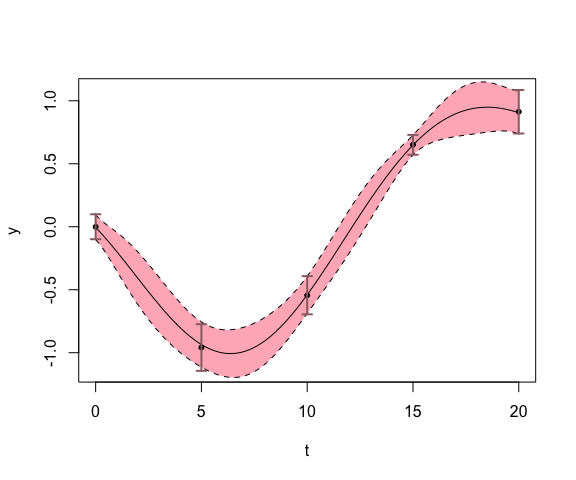Building SQLite database

Once we have had all the results ready, and saved the figures in png format, we can use createDatabase function to create a database which can be used to view the results in the web browser with the help of tigreBrowser. tigreBrowser can be used to display the GP profiles on a browser and to filter them according to provided parameters such as Bayes factors. tigreBrowser is available on GitHub and can be installed with the command:

python setup.py install –user.

Following is a simplified example to create a database containing the provided parameters (Bayes factors and fold changes) and the figures for the genes geneA and geneB:

Note: Please name the figures starting with their corresponding identifiers followed by an underscore and the type of the figure. Specifying the type of the figures allows to display multiple figures for each item.

Once the database is created, it can be viewed with the command:

python tigreServer.py -d database_name.sqlite.

Applications

RNA-seq transcript expression analysis using BitSeq

Sample data

For demonstrating the usage of the functions with examples, we will be using a small sample data from an RNA-seq time series experiment which was introduced by Honkela et al., 2015 and also analysed by Topa and Honkela, 2016. The sample data set, named RNAseqDATA, contains mean and standard deviation information on the expression levels of 5 transcripts (which were originated from 2 genes) at 10 time points (0, 5, 10, 20, 40, 80, 160, 320, 640, 1280 mins) for three settings: “gene”, “abstr”" (absolute transcript), and “reltr”" (relative transcript) expression levels. In addition, the fields “gene_mapping”" and “time_mapping”" include information which is useful to match the genes with transcripts and the time points with data files, respectively. In order to load the data set, type:

If one is interested in getting this data structure from raw BitSeq output files himself, he may use the bitseq_rnaSeqData function:

Assuming BitSeq output files at different time points are named as “t0000.rpkm”, “t0005.rpkm”, “t0010.rpkm”, “t0020.rpkm”, “t0040.rpkm”, “t0080.rpkm”, “t0160.rpkm”, “t0320.rpkm”, “t0640.rpkm”, “t1280.rpkm” and the transcript information file is named as “example_tr”, the following should be able to produce the data structure we have presented here.

Fitting the models

From now on, let us continue with the gene-level data although one can simply perform the same with reltr and abstr levels as well. The function bitseq_fitGPs can be used to fit two GP models to each gene and compute the log Bayes factors:

If one is interested in saving the results into files, one should remember to specify the file names for fileName_logBF, fileName_ModelParams, fileName_NullModelParams and input them as arguments in the bitseq_fitGPs function:

Visualizing the models

Having the GP models fitted to the genes, one can plot the GP profile of a specified gene with the function bitseq_plotGP. For example, the GP profile of the gene ARAP2 shown below can be obtained by the following codes: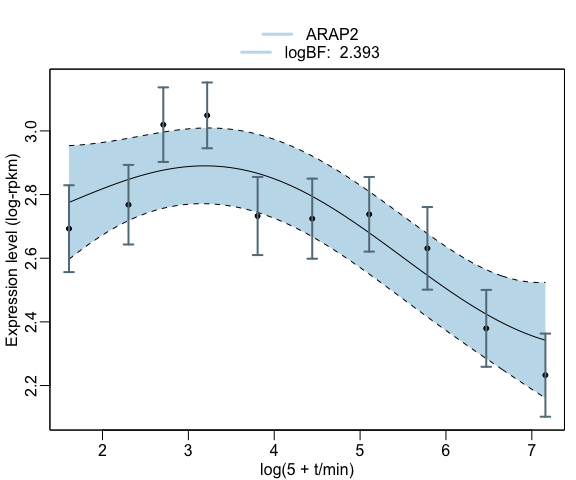Fitted GP model for the overall gene expression levels

In order to save the figure, one can also specify the figure name in plotName option:

The input multi determines whether multiple plots (multi=1) or only a single plot (multi=0) will be plotted on the same figure. For example, if we would like to plot the GP profiles of all the transcripts of ARAP2 gene, we can display all on the same plot by settingmulti` to 1. Let’s try this for absolute transcript expression levels of ARAP2 gene and obtain the figure: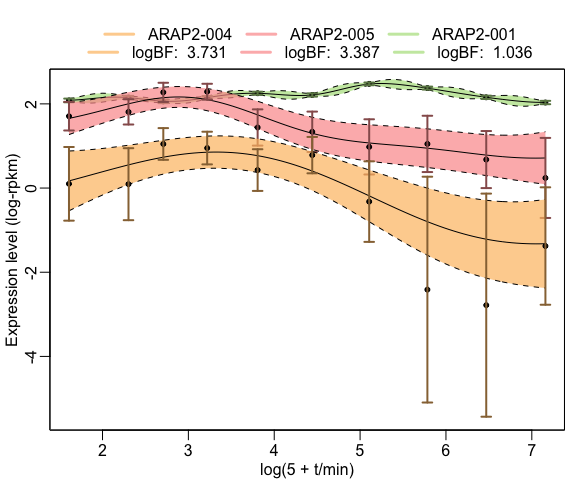Fitted GP model for the absolute transcript expression levels

Let us also do the same for relative transcript expression levels and obtain the corresponding figure: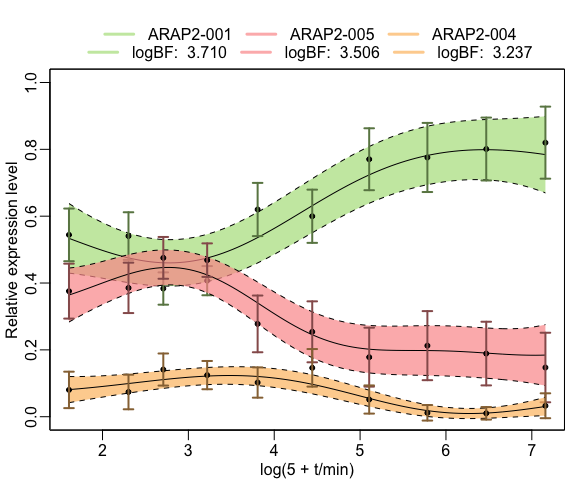Fitted GP model for the relative transcript expression levels

Quantitative analysis of population sequencing data

In Topa et al., 2015, we developed a GP-based method BBGP - beta binomial Gaussian process - for modeling the SNP frequencies in fruit fly populations across several generations in an experimental evolution study. The same method can in principle be used to analyse any population sequencing data where one is interested in the proportion of reads aligning to a specific location that contain a specific feature (such as a SNP).

Sample data

Here we will use a small sample data set named snpData. This sample data set contains 5 replicates of counts and sequencing depth information for 5 SNPs at the generations (0, 10, 20, 30, 40, 50, 60). In order to load the data set, run the command:

If one is interested in getting this data structure from raw data files which have the same format as the synchronized files used by PoPoolation2, (s)he may use the bbgp_snpData function.

Fitting the models

Given the counts and sequencing depth levels, we can use get_bbgpMeanStd function in order to get the posterior means and standard deviations of the frequencies using a beta binomial model with parameters $$\alpha$$ and $$\beta$$ set to 1.

Then, we can perform our GP-based test with apply_gpTest function:

Visualizing the models

Once we have fitted the GP model, we can visualize it using the plotGP function and obtain the figure: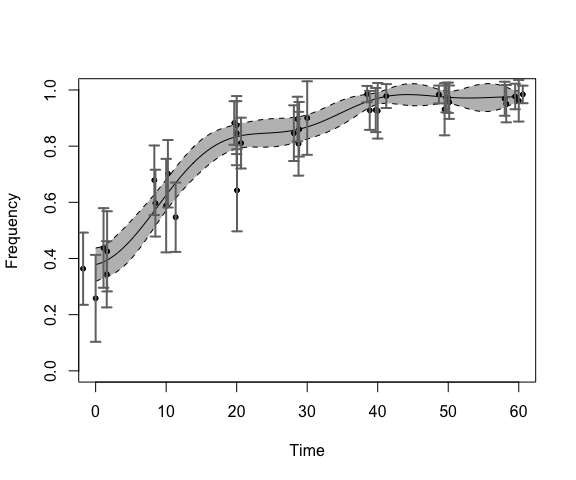Fitted GP model of the SNP frequencies across generations

jitterx option can be used to jitter the observations measured at same time points for clearer visualisation.

References

• Glaus P, Honkela A, Rattray M. Identifying differentially expressed transcripts from RNA-seq data with biological variation. Bioinformatics. 2012;28(13):1721–1728.

• Honkela A, Gao P, Ropponen J, Rattray M, Lawrence ND. tigre: Transcription factor Inference through Gaussian process Reconstruction of Expression for Bioconductor. Bioinformatics. 2011;27(7):1026–1027.

• Kalaitzis AA, Lawrence ND. A Simple Approach to Ranking Differentially Expressed Gene Expression Time Courses through Gaussian Process Regression. BMC Bioinformatics. 2011;12(1):180.

• Kofler, R, Pandey RV, Schlötterer C. PoPoolation2: identifying differentiation between populations using sequencing of pooled DNA samples (Pool-Seq). Bioinformatics. 2011;27(24):3435-3436.

• Rasmussen CE, Williams CKI. Gaussian Processes for Machine Learning. Cambridge, MA: The MIT Press; 2006.

• Topa H, Jónás A, Kofler R, Kosiol C, Honkela A. Gaussian process test for high-throughput sequencing time series: application to experimental evolution. Bioinformatics. 2015;31(11):1762–1770.

• Topa H, Honkela A. Analysis of differential splicing suggests different modes of short-term splicing regulation. Bioinformatics. 2016;32(12):i147–i155.